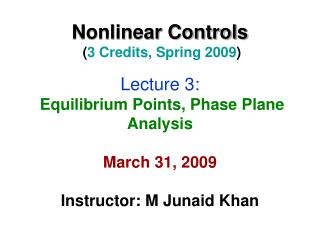DownloadDownload PresentationOutline Home Work Phase Plane Analysis Phase Portraits Symmetry in Phase Plane Portraits

# Outline Home Work Phase Plane Analysis Phase Portraits Symmetry in Phase Plane Portraits

Download Presentation## Outline Home Work Phase Plane Analysis Phase Portraits Symmetry in Phase Plane Portraits

- - - - - - - - - - - - - - - - - - - - - - - - - - - E N D - - - - - - - - - - - - - - - - - - - - - - - - - - -
##### Presentation Transcript

1. Nonlinear Controls (3 Credits, Spring 2009)Lecture 3: Equilibrium Points, Phase Plane AnalysisMarch 31, 2009Instructor: M Junaid Khan

2. Outline • Home Work • Phase Plane Analysis • Phase Portraits • Symmetry in Phase Plane Portraits • Constructing Phase Portraits • Phase Plane Analysis of Linear Systems • Phase Plane Analysis of Nonlinear Systems • Local Behavior of Nonlinear Systems

3. Phase Plane Analysis • Introduced in the end of 19th century by Henry Poincare • Phase Plane analysis is a graphical method of studying second order nonlinear systems • Basic Idea is to solve 2nd order Diff Eqn graphically • The result is a family of system motion trajectories on 2D plane, called phase plane • Only applicable where 2nd order approximation is possible • Give intuitive insights to nonlinear effects • Applies equally well to the analysis of hard nonlinearities • Fundamental disadvantage is application to 2nd order systems

4. Phase Portraits • Phase Plane method is concerned with graphical study of 2nd order systems described by:

5. Phase Portraits Example Solution

6. Phase Portraits A major class of nonlinear systems can be described by: In the state space form

7. Singular Points A singular point is an equilibrium point in the phase plane For linear systems, there is usually only one singular point, while nonlinear systems often have more than one isolated singular point Example

8. This systems has two equilibrium points

9. Phase Plane Method can also be applied to the analysis of first order systems Example There are three singular points

10. Symmetry in Phase Plane Portraits

11. Symmetry in Phase Plane Portraits

12. Constructing Phase Portraits Two methods: Analytical Method and Isocline Method Analytical Method requires analytical solution of the differential equations describing the system Isocline Method is a graphical method, applied to those systems which cannot be solved analytically

13. Constructing Phase Portraits Analytical Method: Refer to slide 5 for the example

14. Constructing Phase Portraits Analytical Method:

15. Constructing Phase Portraits Analytical Method: Remark

16. Constructing Phase Portraits Analytical Method:

17. Constructing Phase Portraits Analytical Method:

18. Constructing Phase Portraits Analytical Method:

19. Constructing Phase Portraits Analytical Method:

20. Constructing Phase Portraits The method of Isoclines: At a point in the phase plane, the slope of the tangent to the trajectory can be given by: An isocline is defined to be the locus of the points with a given tangent slope:

21. Constructing Phase Portraits The method of Isoclines: Example The slope of the trajectories is:

22. Constructing Phase Portraits The method of Isoclines: Example The slope of the trajectories is:

23. Constructing Phase Portraits The method of Isoclines: Example Therefore all the points on the curve: will have slope

24. Constructing Phase Portraits The method of Isoclines:

25. Phase Plane Analysis of Linear Systems Differentiation of first equation and substitution in 2nd

26. Phase Plane Analysis of Linear Systems

27. Phase Plane Analysis of Linear Systems

28. Phase Plane Analysis of Linear Systems

29. Phase Plane Analysis of Linear Systems

30. Phase Plane Analysis of Linear Systems

31. Phase Plane Analysis of Linear Systems